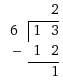# What Is A Improper Fraction?

An improper fraction is a fraction in which the numerator (top number) has a greater value than the denominator (bottom number).
13/6 is an improper fraction to turn it into a mixed number then we divide the numerator by the denominator:The quotient of the above problem is 2 with a remainder of 1.
Now to make our mixed number then we turn the remainder into a fraction:
Put the remainder of 1 over the dividend (The dividend in this case is 6) to make 1/6
So now we have 6 with a remainder of 1/6.
And so 6 1/6 is our mixed number.
thanked the writer.